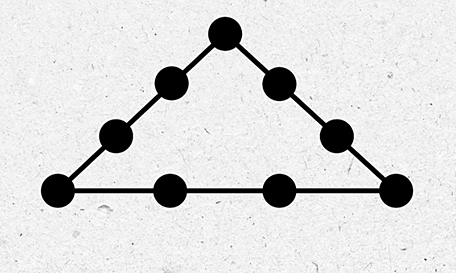Chuyển tới nội dung
Trang chủ » Puzzle to put numbers on the sides of a triangle

# Puzzle to put numbers on the sides of a trianglePuzzle to put numbers on the sides of a triangle

Given the numbers 1 through 9, set the sum of the points on all three sides of the equilateral triangle to 17.

Question 1: Use 4 straight lines to connect the 9 points given below.

Question 2: Join 9 points with three straight lines

Question 3: Use 6 lines to connect 16 dots